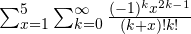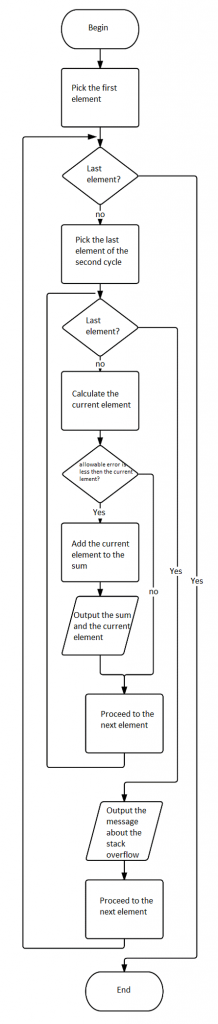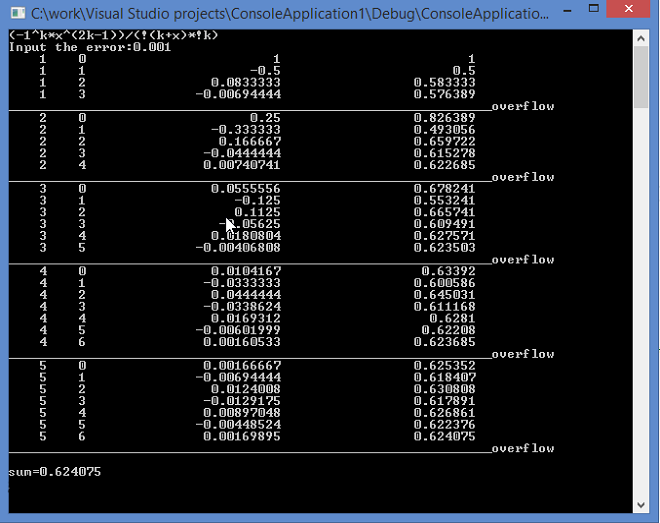# Assignment Example: Cyclic FunctionsBlock scheme of the solution:С++ implementation:


#include<iostream>
#include<math.h>
#include<iomanip>
using namespace std;
float error;
float factorial(int n)
{
float factorial = 1;
for (n; n>0; n--)
factorial *= n;
return factorial;
}
float sum = 0, item;
void main()
{
cout << "(-1^k*x^(2k-1))/(!(k+x)*!k)" << endl;
cout << "Input the error:"; cin >> error;

for (int x = 1; x <= 5; x++)
{
for (int k = 0; k <= 18; k++)//k - float overflowing border
{
item = (pow(-1, k)*pow(x, 2 * k - 1)) / (factorial(k + x)*factorial(k));//calculate item
if (error <= fabs(item))
{
sum += item;//summing items
cout << setw(5) << x << setw(5) << k << setw(25) << item << setw(25) << sum << endl;//intervals & output
}
}
cout << "______________________________________________________________overflow" << endl;
}
cout << endl << "sum=" << sum << endl;//output sum
system("pause");//pause console
}


Screenshot of the working program:## Assignments Homework Help from the Experts

This assignment example was completed by one of experts from AssignmentShark, as well as previously posted one example of assignment on cycles with conditional statement. Due to rigorous recruitment and selection process we work only with the best experts. Each of them is well-qualified and has vast experience. Also, our experts are capable of completing even the most complex tasks. Don’t believe us? Check this on your own.

Fill the order form, choose the most appropriate expert to request do my programming assignment help and you will get the task even better than our assignment example. AssignmentShark is absolutely safe service. We do our best to keep your privacy. Your deadline is looming closer by the minute! Do not hesitate to ask online assignment help!